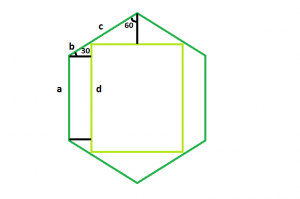# Largest Square that can be inscribed within a hexagon

Given a regular hexagonof side length a, the task is to find the area of the largest square that can be inscribed within it.

Examples:

Input: a = 6
Output: 57.8817

Input: a = 8
Output: 102.901## Recommended: Please try your approach on {IDE} first, before moving on to the solution.

Approach: The square we will derive will have the same centre and axes of the hexagon. This is because the square will become smaller if we will rotate it.

The sides of the hexagonal are equal i.e. a = b + c.
Now, let d be the length of the side of the inscribed square,
Then the top side of the square, d = 2 * c * sin(60).
And, the left side of the square, d = a + 2 * b * sin(30).
Substituting for c, d = 2 * (a – b) * sin(60).
Now taking d and re-arranging, we get, b / a = (2 * sin(60) – 1) / (2 * (sin(30) + sin(60)))
So, b / a = 2 – √3
Now, substituting the relation of b and a in the left hand side equation of square, we get,
d / a = 3 – √3 i.e. d / a = 1.268
Therefore, d = 1.268 * a

Below is the implementation of the above approach:

## C++

 `// C++ program to find the area of the largest square ` `// that can be inscribed within the hexagon ` `#include ` `using` `namespace` `std; ` ` `  `// Function to find the area ` `// of the square ` `float` `squareArea(``float` `a) ` `{ ` ` `  `    ``// Side cannot be negative ` `    ``if` `(a < 0) ` `        ``return` `-1; ` ` `  `    ``// Area of the square ` `    ``float` `area = ``pow``(1.268, 2) * ``pow``(a, 2); ` `    ``return` `area; ` `} ` ` `  `// Driver code ` `int` `main() ` `{ ` `    ``float` `a = 6; ` `    ``cout << squareArea(a) << endl; ` `    ``return` `0; ` `} `

## Java

 `// Java program to find the area of the largest square ` `// that can be inscribed within the hexagon ` `class` `Solution { ` `    ``// Function to find the area ` `    ``// of the square ` `    ``static` `float` `squareArea(``float` `a) ` `    ``{ ` ` `  `        ``// Side cannot be negative ` `        ``if` `(a < ``0``) ` `            ``return` `-``1``; ` ` `  `        ``// Area of the square ` `        ``float` `area = (``float``)(Math.pow(``1.268``, ``2``) * Math.pow(a, ``2``)); ` `        ``return` `area; ` `    ``} ` ` `  `    ``// Driver code ` `    ``public` `static` `void` `main(String args[]) ` `    ``{ ` `        ``float` `a = ``6``; ` `        ``System.out.println(squareArea(a)); ` `    ``} ` `} ` ` `  `// This code is contributed by Arnab Kundu `

## Python3

 `# Python program to find the area of the largest square ` `# that can be inscribed within the hexagon ` ` `  `# Function to find the area ` `# of the square ` `def` `squareArea(a): ` ` `  `    ``# Side cannot be negative ` `    ``if` `(a < ``0``): ` `        ``return` `-``1``; ` ` `  `    ``# Area of the square ` `    ``area ``=` `(``1.268` `*``*` `2``) ``*` `(a ``*``*` `2``); ` `    ``return` `area; ` ` `  `# Driver code ` `a ``=` `6``; ` `print``(squareArea(a)); ` ` `  `# This code contributed by PrinciRaj1992  `

## C#

 `// C# program to find the area of the largest square ` `// that can be inscribed within the hexagon ` `using` `System; ` `class` `Solution { ` `    ``// Function to find the area ` `    ``// of the square ` `    ``static` `float` `squareArea(``float` `a) ` `    ``{ ` ` `  `        ``// Side cannot be negative ` `        ``if` `(a < 0) ` `            ``return` `-1; ` ` `  `        ``// Area of the square ` `        ``float` `area = (``float``)(Math.Pow(1.268, 2) * Math.Pow(a, 2)); ` `        ``return` `area; ` `    ``} ` ` `  `    ``// Driver code ` `    ``public` `static` `void` `Main() ` `    ``{ ` `        ``float` `a = 6; ` `        ``Console.WriteLine(squareArea(a)); ` `    ``} ` `} ` ` `  `// This code is contributed by  anuj_67.. `

## PHP

 ` `

Output:

```57.8817
```

My Personal Notes arrow_drop_upCheck out this Author's contributed articles.

If you like GeeksforGeeks and would like to contribute, you can also write an article using contribute.geeksforgeeks.org or mail your article to contribute@geeksforgeeks.org. See your article appearing on the GeeksforGeeks main page and help other Geeks.

Please Improve this article if you find anything incorrect by clicking on the "Improve Article" button below.

Article Tags :
Practice Tags :

Be the First to upvote.

Please write to us at contribute@geeksforgeeks.org to report any issue with the above content.